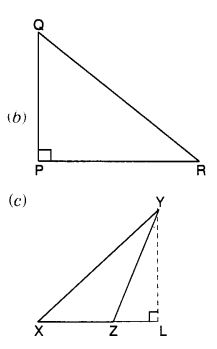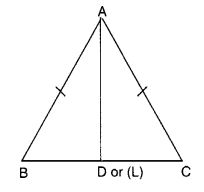NCERT Solutions for Class 7 Maths Chapter 6 The Triangle and its Properties Ex 6.1 are part of NCERT Solutions for Class 7 Maths. Here we have given NCERT Solutions for Class 7 Maths Chapter 6 The Triangle and its Properties Ex 6.1.

 Board CBSE Textbook NCERT Class Class 7 Subject Maths Chapter Chapter 6 Chapter Name The Triangle and its Properties Exercise Ex 6.1 Number of Questions Solved 3 Category NCERT Solutions

## NCERT Solutions for Class 7 Maths Chapter 6 The Triangle and its Properties Ex 6.1

Question 1.
In ∆PQR, D is the mid-point of $$\overline { QR }$$.Solution:
$$\overline { PM }$$ is the altitude.
PD is the median.
No! QM ≠ MR.

Question 2.
Draw rough sketches for the following:

(a) In ∆ ABC, BE is a median.
(b) In ∆ PQR, PQ and PR are altitudes of the triangle.
(c) In ∆ XYZ, YL is an altitude in the exterior of the triangle.

Solution:Question 3.
Verify by drawing a diagram if the median and altitude of an isosceles triangle can be the same.
Solution: